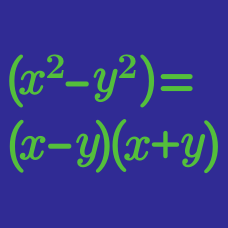Algebra

# Factoring Polynomials Using Identities

Which of the following must be a factor of

$x^3 + 8 ?$

Note: $a^3 + b^3 = ( a + b ) ( a^2 - ab + b^2 ) .$

Using the identity $a^2 - b^2 = (a-b) ( a+b)$, which of the following must be a factor of

$x^4 + 2500 ?$

If $a$ and $b$ are positive numbers and $x^4-38x^2y^2+y^4=(x^2+axy-y^2)(x^2-bxy-y^2),$ what is the value of $a+b$?

Suppose $a$, $b$, $c$ and $d$ are all non-positive integers such that the following is an algebraic identity in $x$: $(x-a)^2(x+a)^2=x^4+bx^3+cx^2+dx+256.$ What is the value of $-a-b-c-d$?Which of the following is a factor of $x^4 - 81$?

×